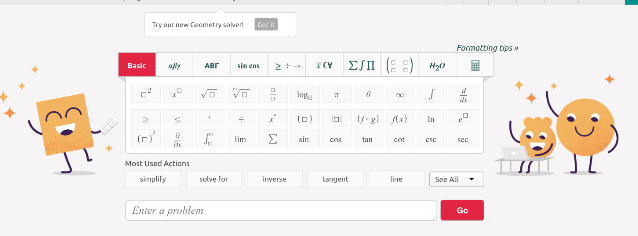Symbolab Calculator and Math Solve- Full Review

Symbolab is an educational math tool that allows you to discover, practice, and learn math problems using scientific calculators and symbols. It is both an equation search and math solver. Students from middle school through college will especially find it helpful. They can access detailed step by step solutions to any math problem related to algebraic, trigonometric and calculus topics.
Students can use Symbolab to not only solve math problems (e.g., Integrals, Equations, derivatives, limits, etc.) but to also understand processes used to reach solutions to math problems. It acts like a private math tutor available 24/7.Image credit: Symbolab.com

The strength of Symbolab resides in its calculating powers. It offers access to a plethora of sophisticated calculators including ‘equations, simultaneous equations, inequalities, integrals, derivatives, limits , tangent line, trigonometric equations, functions and more’.
The purpose, according to Symbolab, is to simplify math learning and ‘make scientific content universally accessible by expanding the searchable data space onto scientific notations, expressions, equations and formulas’.
Symbolab offers the following services:
• Integral Calculator
• Derivative Calculator
• Limit calculator
• Equation Calculator
• Inequality Calculator
• Trigonometry Calculator
• Matrix Calculator
• Functions Calculator
• Series Calculator
• ODE Calculator
• Laplace Transform Calculator
Symbolab graphing calculator allows students to graph functions they like.  Each function has its own colour making it easy for students to identify them. Graphs can be saved as PDFs or printed out. ‘Symbolab’s Graphing Calculator offers a color map, parameter sliders, table that shows the points for each function, graph and grid settings, and function properties.’

Students get practice working on different math problems and quizzes related to the following areas: pre-Algebra, Algebra, Calculus, Trigonometry, Functions, Word problems, Matrices and Vectors.
Each of these topics include a number of sub-topics. Simply select a topic and click on the subtopic you are interested in and use the left side bar to access quizzes and practice problems. Premium subscribers can create custom quizzes to share with others.

Symbalolab notebook provides you with a digital space where you can write and save your notes and math problems. It lets you ‘save any problem and graph, tag and filter, add notes, and share with your friends.’
Teachers can use Symbolab Groups to create virtual classrooms where they can share math problems and graphs with their students, conduct assessments, gather students feedback, and use the analytic reports to access insights on students performance. Watch this video to learn more how to create groups in Symbolab.